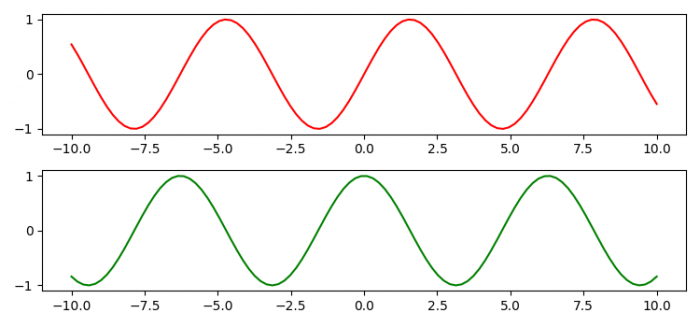# How to combine several matplotlib axes subplots into one figure?

To combine several matplotlib axes subplots into one figure, we can use subplots() method with nrow=2.

## Steps

• Set the figure size and adjust the padding between and around the subplots.
• Create x, y1 and y2 data points using numpy.
• Create a figure and a set of subplots.
• Plot x, y1 and y2 data points using plot() method.
• To display the figure, use show() method.

## Example

import numpy as np
from matplotlib import pyplot as plt

plt.rcParams["figure.figsize"] = [7.50, 3.50]
plt.rcParams["figure.autolayout"] = True

x = np.linspace(-10, 10, 100)

y1 = np.sin(x)
y2 = np.cos(x)

fig, axes = plt.subplots(nrows=2)

line1, = axes.plot(x, y1, color='red')
line2, = axes.plot(x, y2, color='green')

plt.show()

## Output